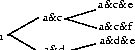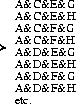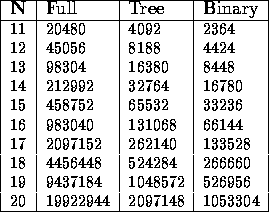Next: Conclusion Up: Reducing Complexity in A Previous: Extracting Sub-Grammars for

Improving the Efficiency of Expansion

Section 4 has proposed techniques which reduce the size of the description which needs to be expanded. However, for large-sized descriptions, the expansion is still complex. This section briefly explores two methods which increase the efficiency of the expansion process. If we can't avoid full expansion, then at least we can make the expansion process more efficient.

``Structure Sharing'' in Expansion

This section assumes a disjunctive description of the following form:

(:and (:xor A B) (:xor C D) (:xor E F) )

Logical form V introduced above was of this form. Much of the pre-processing in the parser involves the DNF-expansion of disjunctions in this form.

Full Expansion

The brute force method for expanding this form involves:

1. Find all combinations of terms, taking one term from each disjunction.
2. Test compatibility of each combination, eliminating combinations which are internally inconsistent.
Step 1 of this process produces the following DNF form:

(:xor (:and A C E) (:and A C F) (:and A D E) (:and A D F) (:and B C E) (:and B C F) (:and B D E) (:and B D F) )

The problem with this approach is with the incompatibility checking -- the same checks will be repeated over and over again. For instance, the incompatibility check between A and C is repeated twice: (:and A C E) and (:and A C F). This repetition occurs for every pair of terms in the conjuncts. The problem gets worse exponentially as we add more disjuncts.

To avoid this redundancy, we need something like a chart in parsing, a method to record the results of each unification and thus avoid repeating any unification.

Unfortunately, DNF expansion is not quite like parsing. We can test the consistency between any two pairs of terms (for instance A and C in the above), but we also need to know about the consistency of terms in combination e.g., the pairs: A&C, A&E and C&E may be consistent, but the combination A&C&E may not be.

The rest of this section describes two techniques which allow some redundancy reduction, sometimes known as structure-sharing.

Tree Organisation of Expansion

The disjunctive description above can easily be re-represented in the form below:

(:and (:and (:xor A B) (:xor C D)) (:xor E F))

The process here involves expanding out the first two disjunctions, eliminating inconsistent results, and then expanding the result out with the next disjunction. The incremental expansion is illustrated in Figure 9.Figure 9: Tree expansion method

This method is more efficient than the full expansion method, since:

• Some terms, such as a&c, a&d etc. are unified only once. However note that terms e and f are still involved in multiple products.
• the failure of a combination of terms early in the unification process eliminates a large number of expansions by the end of the process.

Binary Organisation of Expansion

A third approach aims at maximising the degree of `sharing' unifications in the expansion. The disjunctions in the description are split into pairs, and unified. The results of these unifications are then unified in the same pair-wise manner. This expansion for a conjunction of four disjunctions is shown in Figure 10.Figure 10: Binary expansion method

The advantage of this approach is that we are maximising the amount of structure-sharing in the unification.

Comparison of Expansion Approaches

We compared the number of unifications which take place using each of these methods for various numbers of disjunctions (all disjunctions having two disjuncts).

One can see from Table 1 that the worst-case score for the full expansion method is far worse than the other methods. It is not a practical method.Table 1: Worst-case comparison

Comparing the worst-case for the `tree' and the `binary' expansion method, we see that the binary method clearly comes out better, by around 50%.

We also did a simulation to check an average case score, since the worst-case score doesn't take into account that many later unifications are avoided when early unification proves inconsistent. We found that while the binary method still seems superior, in some instances the tree method requires fewer unifications. More work is needed here.

Ordering Incompatible Disjunctions First

When using either the Tree or Binary expansion methods, fewer unifications will be required if we place the disjunctions with the greatest chance of inconsistency first. In a sense, we are pruning inconsistent branches of the expansion tree `at the root'.

In the systemic parser, several heuristics have been used to group disjunctions which are most likely to produce the fewest cross-products, and perform these first.

One possible method for utilising this phenomenon is:

1. Separate the disjunctions into sub-sets which maximise likelihood of incompatibility between rules inside the sub-expressions.
2. Expand out the disjunctions inside each sub-set. The results of each sub-set are cached so they need only be expanded once.
3. Expand out the results of (2) against each other.

Avoiding Expansion of Incompatible Terms

Sometimes, it is possible to tell without full unification that a set of rules will not unify with another set. For instance, assume a larger grammar than the one we have been using, a grammar which includes clauses, nominal-groups, prepositional phrases, adverbial phrases and words. These categories are all types in the system network, just like any other types.

Since these types won't unify with each other, we can also know that types which inherit from one of these basic types will not unify with the the sub-types of another basic type. We thus do not need to try to unify descriptions which differ in their basic type. If we split any disjunctive description into sub-components for each basic type, we know a priori that there is no unification between these sub-components.

Before trying any of the expansion techniques outlined in this paper, the whole grammar is segmented into sub-descriptions, one for each of these basic types. The complexity of the expansion of each of these sub-grammars is less than for the grammar as a whole.

Other principles can be used to locate sets of rules which will not unify. These can be applied also.Next: Conclusion Up: Reducing Complexity in A Previous: Extracting Sub-Grammars for

Mick O'Donnell
Fri Jan 26 19:21:43 GMT 1996